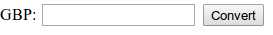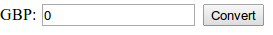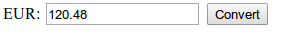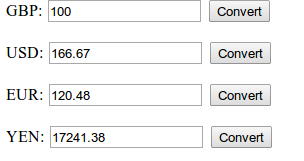# MVC in PHP tutorial part 2: Real world program

You may be interested in my newer article: Immutable MVC in PHP (Part 2) - Immutable CRUD application which shows similar concepts but demonstrates a CRUD application with a database.

## Introduction

This is a step by step tutorial for implementing MVC in PHP in a real world application. I've posted many articles which are hypothetical and full of the theory behind MVC but today here's something for the pragmatists. Something you can see be developed one step at a time to produced a real application: The application will be a currency converter. It will convert from a chosen currency to several others and display the values in each currency.

This example will show a bare-bones, lightweight MVC application and finish by highlighting some of the problems which arise when using this approach. At the end you'll understand the proper separation of concers of MVC and at the same time, be aware of the limitations of assuming the domain model and MVC model are the same thing.

## Converting currencies - The Model

Before even starting worrying about MVC or the structure of the application, the base problem needs to be solved: doing the currency conversion. For this I can create a simple class:

``` class CurrencyConverter {     private \$baseValue = 0;     private \$rates = [         'GBP' => 1.0,         'USD' => 0.6,         'EUR' => 0.83,         'YEN' => 0.0058     ];     public function get(\$currency) {         if (isset(\$this->rates[\$currency])) {             \$rate = 1/\$this->rates[\$currency];             return round(\$this->baseValue * \$rate, 2);         }         else return 0;     }     public function set(\$amount, \$currency = 'GBP') {         if (isset(\$this->rates[\$currency])) {             \$this->baseValue = \$amount * \$this->rates[\$currency];         }     } } ```

As you can see, this stores the exchange rates relative to GBP (British Pounds) and performs the conversion. It stores a specific amount always stored as the value in GBP. However, the base value is not directly accessible. The maths involved and implementation of this class isn't important, what is important is that the class can be used to do conversions like this:

``` \$currencyConverter = new CurrencyConverter; \$currencyConverter->set(100, 'GBP'); echo '100 GBP is:'; echo \$currencyConverter->get('USD') . ' USD / '; echo \$currencyConverter->get('EUR') . ' EUR / '; echo \$currencyConverter->get('YEN') . ' YEN'; ```

Which will output:

``` 100 GBP is: 166.67 USD / 120.48 EUR / 17241.38 YEN ```

Alternatively, you could convert USD to other currencies using:

``` \$currencyConverter = new CurrencyConverter; \$currencyConverter->set(100, 'USD'); echo '100 USD is: '; echo \$currencyConverter->get('GBP') . ' GBP / '; echo \$currencyConverter->get('EUR') . ' EUR / '; echo \$currencyConverter->get('YEN') . ' YEN'; ```

Which will print:

``` 100 USD is: 60 GBP / 72.29 EUR / 10344.83 YEN ```

This converter works without any knowledge of how it will be used, the architecture it might be used in and can work as its own standalone component.

This is the Domain Model. For this part of the tutorial, I will use the Domain Model as the Model in MVC. The next article will discuss moving beyond this approach and the limitations of it.

## The View

Now that the domain model is working successfully the next step is to create the View. Since the currency converter will require the user to type in a value in the currency they're converting from an input box will be required as well as a submit button for them to press. It's probably worth displaying the "From" currency as well.

The view will need to know which currency it's dealing with. In this instance, we'll start with GBP.

The view might look something like this:

``` class CurrencyConverterView {     private \$currency;     public function __construct(\$currency) {         \$this->currency = \$currency;     }     public function output() {         \$html = '<form action="?action=convert" method="post">'                 .    '<input name="currency" type="hidden" value="' . \$this->currency .'"/>'                 .    '<label>' . \$this->currency .':</label>'                 .    '<input name="amount" type="text" value=""/>'                 .    '<input type="submit" value="Convert"/>'                 '</form>';         return \$html;     } } ```

Which when instantiated using the code:

``` \$view = new CurrencyConverterView('GBP'); echo \$view->output(); ```

Will display the following:At the moment it's not actually displaying anything meaningful as it doesn't know about the converter. The next step is to pass it the model, and have it display the value which is in the model:

``` class CurrencyConverterView {     private \$converter;     private \$currency;     public function __construct(CurrencyConverter \$converter, \$currency) {         \$this->converter = \$converter;         \$this->currency = \$currency;     }     public function output() {         \$html = '<form action="?action=convert" method="post">'                 .    '<input name="currency" type="hidden" value="' . \$this->currency .'"/>'                 .    '<label>' . \$this->currency .':</label>'                 .    '<input name="amount" type="text" value="' . \$this->converter->get(\$this->currency) . '"/>'                 .    '<input type="submit" value="Convert"/>'                 '</form>';         return \$html;     } } ```

The view can now display currencies. Using the code:

``` \$currencyConverter = new CurrencyConverter; \$view = new CurrencyConverterView(\$currencyConverter, 'GBP'); echo \$view->output(); ```This is not very informative. But we can test it using by setting a value in the model and displaying a different currency:

``` \$currencyConverter = new CurrencyConverter; \$currencyConverter->set('100', 'GBP'); \$view = new CurrencyConverterView(\$currencyConverter, 'EUR'); echo \$view->output(); ```Which is correctly displaying the EUR value of 100 GBP. On its own, this isn't very useful. However, because MVC is all about reusability, the View can be reused with the other currencies:

``` \$currencyConverter = new CurrencyConverter; \$currencyConverter->set('100', 'GBP'); \$gbpView = new CurrencyConverterView(\$currencyConverter, 'GBP'); echo \$gbpView->output(); \$usdView = new CurrencyConverterView(\$currencyConverter, 'USD'); echo \$usdView->output(); \$eurView = new CurrencyConverterView(\$currencyConverter, 'EUR'); echo \$eurView->output(); \$yenView = new CurrencyConverterView(\$currencyConverter, 'YEN'); echo \$yenView->output(); ```

Which will output:By reusing the view multiple times, the data from the model can be shown in each currency.

## Enabling user input - The Controller

The final piece of the puzzle is making the "Convert" button work. This is the last step in MVC and why Model-View-Controller is MVC instead of CVM. The controller needs to take user input and update the model in some way. The controller could look like this:

``` class CurrencyConverterController {     private \$currencyConverter;     public function __construct(CurrencyConverter \$currencyConverter) {         \$this->currencyConverter = \$currencyConverter;     }     public function convert(\$request) {         if (isset(\$request['currency']) && isset(\$request['amount'])) {             \$this->currencyConverter->set(\$request['amount'], \$request['currency']);         }     } } ```

This uses the form field names which we already created in the view. In order to make the controller reusable and testable, it doesn't use \$_POST directly but takes an array which in this example is passed in as an argument.

The application can now be initialised with the following code:

``` \$model = new CurrencyConverter(); \$controller = new CurrencyConverterController(\$model); //Check for presence of \$_GET['action'] to see if a controller action is required if (isset(\$_GET['action'])) \$controller->{\$_GET['action']}(\$_POST); \$gbpView = new CurrencyConverterView(\$model, 'GBP'); echo \$gbpView->output(); \$usdView = new CurrencyConverterView(\$model, 'USD'); echo \$usdView->output(); \$eurView = new CurrencyConverterView(\$model, 'EUR'); echo \$eurView->output(); \$yenView = new CurrencyConverterView(\$model, 'YEN'); echo \$yenView->output(); ```

And the conversion will take place based on which convert button you press. Try it yourself or view the entire source code

## Conclusion

This is an example of using MVC to solve a real world problem. Some of the key things to note about implementing MVC are that, for this application:

• The same view class is instantiated 4 times
• The controller is only instantiated once and used by all the views
• The view can be used without a controller (but user actions do not work)
• The controller doesn't interract with the view at all
• Because the controller isn't feeding information to the view or directing program flow, the controller doesn't need to be instantiated separately for each instance of the view

As noted earlier, there are a few limitations with this approach because the Domain Model has been used as the MVC model. These will be discussed in detail in the next article.